Next: Evaluation of XML retrieval Up: XML retrieval Previous: Challenges in XML retrieval   Contents   Index

# A vector space model for XML retrieval

In this section, we present a simple vector space model for XML retrieval. It is not intended to be a complete description of a state-of-the-art system. Instead, we want to give the reader a flavor of how documents can be represented and retrieved in XML retrieval.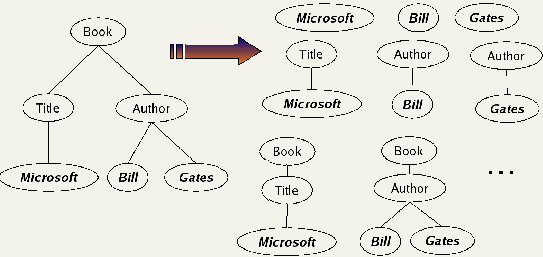To take account of structure in retrieval in Figure 10.4 , we want a book entitled Julius Caesar to be a match for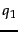and no match (or a lower weighted match) for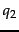. In unstructured retrieval, there would be a single dimension of the vector space for Caesar. In XML retrieval, we must separate the title word Caesar from the author name Caesar. One way of doing this is to have each dimension of the vector space encode a word together with its position within the XML tree.

Figure 10.8 illustrates this representation. We first take each text node (which in our setup is always a leaf) and break it into multiple nodes, one for each word. So the leaf node Bill Gates is split into two leaves Bill and Gates. Next we define the dimensions of the vector space to be lexicalized subtrees of documents - subtrees that contain at least one vocabulary term. A subset of these possible lexicalized subtrees is shown in the figure, but there are others - e.g., the subtree corresponding to the whole document with the leaf node Gates removed. We can now represent queries and documents as vectors in this space of lexicalized subtrees and compute matches between them. This means that we can use the vector space formalism from Chapter 6 for XML retrieval. The main difference is that the dimensions of vector space in unstructured retrieval are vocabulary terms whereas they are lexicalized subtrees in XML retrieval.

There is a tradeoff between the dimensionality of the space and accuracy of query results. If we trivially restrict dimensions to vocabulary terms, then we have a standard vector space retrieval system that will retrieve many documents that do not match the structure of the query (e.g., Gates in the title as opposed to the author element). If we create a separate dimension for each lexicalized subtree occurring in the collection, the dimensionality of the space becomes too large. A compromise is to index all paths that end in a single vocabulary term, in other words, all XML-contextterm pairs. We call such an XML-contextterm pair a structural term and denote it by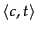: a pair of XML-context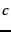and vocabulary term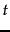. The document in Figure 10.8 has nine structural terms. Seven are shown (e.g., "Bill" and Author#"Bill") and two are not shown: /Book/Author#"Bill" and /Book/Author#"Gates". The tree with the leaves Bill and Gates is a lexicalized subtree that is not a structural term. We use the previously introduced pseudo-XPath notation for structural terms.

As we discussed in the last section users are bad at remembering details about the schema and at constructing queries that comply with the schema. We will therefore interpret all queries as extended queries - that is, there can be an arbitrary number of intervening nodes in the document for any parent-child node pair in the query. For example, we interpret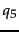in Figure 10.7 as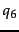.

But we still prefer documents that match the query structure closely by inserting fewer additional nodes. We ensure that retrieval results respect this preference by computing a weight for each match. A simple measure of the similarity of a path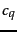in a query and a path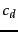in a document is the following context resemblance function CR: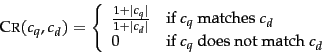(52)

where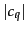and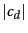are the number of nodes in the query path and document path, respectively, andmatchesiff we can transformintoby inserting additional nodes. Two examples from Figure 10.6 are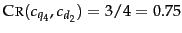and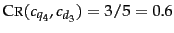where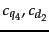and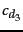are the relevant paths from top to leaf node in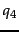,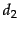and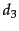, respectively. The value of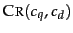is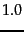if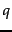and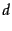are identical.

The final score for a document is computed as a variant of the cosine measure (Equation 24, page 6.3.1 ), which we call SIMNOMERGE for reasons that will become clear shortly. SIMNOMERGE is defined as follows: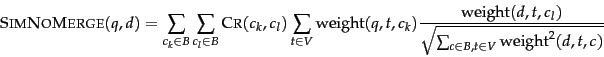(53)

where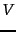is the vocabulary of non-structural terms;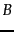is the set of all XML contexts; and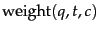and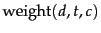are the weights of termin XML contextin queryand document, respectively. We compute the weights using one of the weightings from Chapter 6 , such as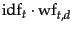. The inverse document frequency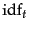depends on which elements we use to compute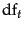as discussed in Section 10.2 . The similarity measure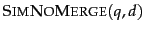is not a true cosine measure since its value can be larger than 1.0 (Exercise 10.7 ). We divide by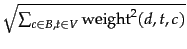to normalize for document length (Section 6.3.1 , page 6.3.1 ). We have omitted query length normalization to simplify the formula. It has no effect on ranking since, for a given query, the normalizer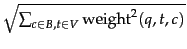is the same for all documents.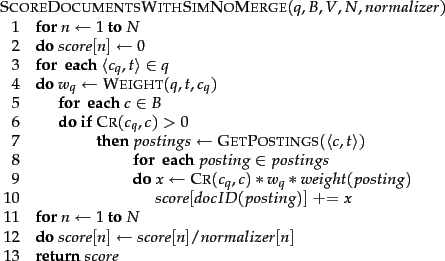The algorithm for computing SIMNOMERGE for all documents in the collection is shown in Figure 10.9 . The array normalizer in Figure 10.9 containsfrom Equation 53 for each document.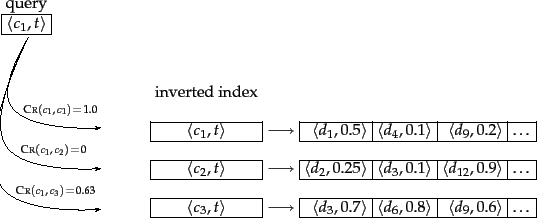We give an example of how SIMNOMERGE computes query-document similarities in Figure 10.10 .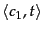is one of the structural terms in the query. We successively retrieve all postings lists for structural terms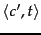with the same vocabulary term. Three example postings lists are shown. For the first one, we have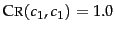since the two contexts are identical. The next context has no context resemblance with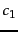: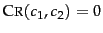and the corresponding postings list is ignored. The context match ofwith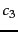is 0.63>0 and it will be processed. In this example, the highest ranking document is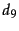with a similarity of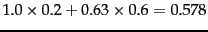. To simplify the figure, the query weight ofis assumed to be 1.0.

The query-document similarity function in Figure 10.9 is called SIMNOMERGE because different XML contexts are kept separate for the purpose of weighting. An alternative similarity function is SIMMERGE which relaxes the matching conditions of query and document further in the following three ways.

• We collect the statistics used for computingandfrom all contexts that have a non-zero resemblance to(as opposed to just fromas in SIMNOMERGE). For instance, for computing the document frequency of the structural term atl#"recognition", we also count occurrences of recognition in XML contexts fm/atl, article//atl etc.
• We modify Equation 53 by merging all structural terms in the document that have a non-zero context resemblance to a given query structural term. For example, the contexts /play/act/scene/title and /play/title in the document will be merged when matching against the query term /play/title#"Macbeth".
• The context resemblance function is further relaxed: Contexts have a non-zero resemblance in many cases where the definition of CR in Equation 52 returns 0.
See the references in Section 10.6 for details.

These three changes alleviate the problem of sparse term statistics discussed in Section 10.2 and increase the robustness of the matching function against poorly posed structural queries. The evaluation of SIMNOMERGE and SIMMERGE in the next section shows that the relaxed matching conditions of SIMMERGE increase the effectiveness of XML retrieval.

Exercises.

• Consider computing df for a structural term as the number of times that the structural term occurs under a particular parent node. Assume the following: the structural term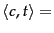author#"Herbert" occurs once as the child of the node squib; there are 10 squib nodes in the collection;occurs 1000 times as the child of article; there are 1,000,000 article nodes in the collection. The idf weight ofthen is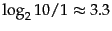when occurring as the child of squib and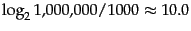when occurring as the child of article. (i) Explain why this is not an appropriate weighting for. Why shouldnot receive a weight that is three times higher in articles than in squibs? (ii) Suggest a better way of computing idf.

• Write down all the structural terms occurring in the XML document in Figure 10.8 .

• How many structural terms does the document in Figure 10.1 yield?Next: Evaluation of XML retrieval Up: XML retrieval Previous: Challenges in XML retrieval   Contents   Index